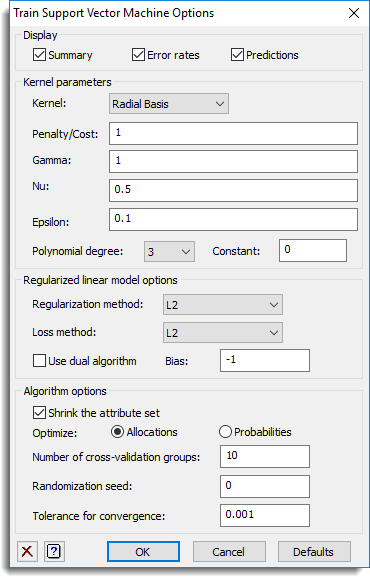1. Home
2. Train Support Vector Machine Options

# Train Support Vector Machine Options

Use this to select the output to be generated when training a support vector machine, and options used in the analysis.## Display

Specifies which items of output are to be displayed in the Output window.

 Summary Summary of the support vector machine fitted. Error rates The error rates for the classification to groups or the mean-squared error between the predictions and y-variate for a regression method Predictions Estimated groups or y-variate from the fitted support vector machine.

## Kernel

The kernel to use for the support vector machines. This is not used for the linear or Cramer & Singer methods:

 Radial basis Use the radial basis function exp(-gamma*|u – v|**2) to measure distance between points u and v. Linear Use the linear function u’v to measure distance between points u and v Polynomial Use the polynomial function gamma*(u’v + c)**d to measure distance between points u and v Sigmoid Use the sigmoid function tanh(gamma*u’v + c) to measure distance between points u and v

## Penalty/cost

The penalty applied to the sum of distances for the points on the wrong side of the boundary. The larger this is, the more weight that is applied to points that are on the wrong side of the discrimination boundaries. This may be a single number, a scalar, a variate, or a space or comma separated list of numbers. If more than one value is provided, they are all tried and the one which minimizes the error rate is used.

## Gamma

This specifies a value for gamma to control the amount of smoothing applied to the boundaries formed when using non-linear kernels. The larger this number is, the smoother the boundaries will be. This may be a single number, a scalar, a variate, or a space or comma separated list of numbers. If more than one value is provided, they are all tried and the one which minimizes the error rate is used.

## Nu

The penalty applied to having too many support vectors in the Nu support vector classifier and regression methods. The larger this number is, the smaller the number of support vectors that will be used. This may be a single number, a scalar, a variate, or a space or comma separated list of numbers. If more than one value is provided, they are all tried and the one which minimizes the error rate is used.

## Epsilon

The value of epsilon used the support vector regression method. This may be a single number, a scalar, a variate, or a space or comma separated list of numbers. If more than one value is provided, they are all tried and the one which minimizes the error rate is used.

## Constant

This gives the parameters c in the distance function gamma*(u’v + c)**d, when a polynomial kernel is chosen, and tanh(gamma*u’v + c) when a sigmoid kernel is chosen.

## Polynomial degree

When a polynomial kernel is chosen, this gives the parameter d in the distance function gamma*(u’v – c)**d.

## Regularized linear model options

When a linear support vector classifier or regression is chosen, these options control the linear algorithm used.

## Regularization method

This sets the regularization method used with the linear model.

 L2 The regularization method uses the square of the coefficients L1 The regularization method uses the absolute value of the coefficients Logistic The regularization method uses logistic regression

## Loss method

This sets the loss method used with the linear model.

 L2 The loss method uses the square of the error L1 The loss method uses the absolute value of the error

## Use dual algorithm

If this is selected, the dual algorithm will be used. This may be faster if there are more attributes than cases.

## Bias

This provides a space to supply a bias for the Regularized linear support vector classifier method. If this is -1, then no bias is used. You use a bias to attempt to achieve a more optimal discrimination between groups. When the bias is set to a non-negative value, an extra constant attribute is added to the end of each individual. This extra attribute controls the origin of the separating hyper-plane (the origin is where all attributes have value of 0). A bias of 0 forces the separating hyper-plane to go through the origin, and a positive value moves the plane away from the origin. The bias thus acts as a tuning parameter, that changes the hyper-plane’s origin. You can try different biases to attempt to improve the discrimination.

## Shrink the attribute set

The set of attributes is shrunken to eliminate unneeded attributes, and so speed up the algorithm.

## Optimise

This option controls what criterion is used for optimization

 Allocations The number of correct allocations is optimized Probability The sum of the probabilities of the correct allocations is optimized

## Number of cross-validation groups

For the cross-validation, this gives the number of groups the data will be allocated to. Each group is then left out of the analysis and predicted from the remaining groups.

## Randomization seed

This provides a space to supply a seed to initialize the random number generation used for cross-validation. Using zero initializes this from the computer’s clock, but specifying an nonzero value gives a repeatable analysis.

## Tolerance for convergence

This provides a space to supply a small positive value used to decide when the optimization of the support vector machine has converged. Increasing this value will give a faster convergence but the support vector machine may be further from the optimum. Decreasing the value may give an improved support vector machine but it will take longer to fit.